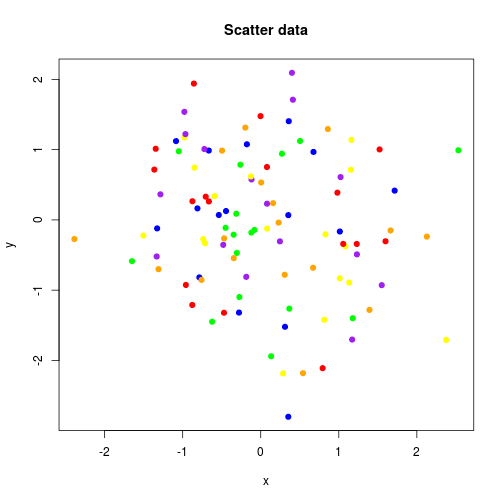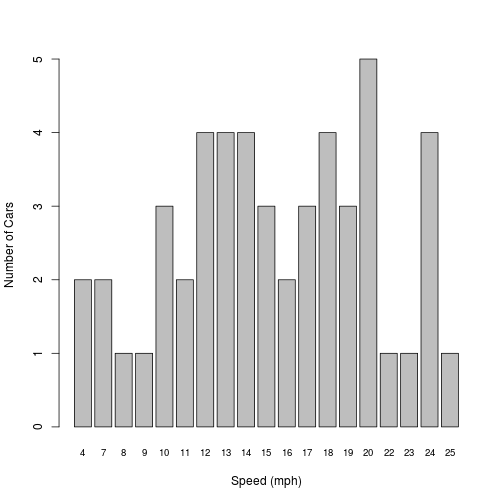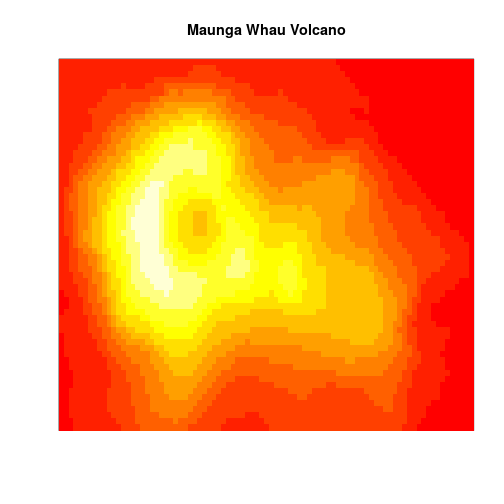# Static Content

The interactivity of the AnalysisPageServer front end (rollover, zoom, tag, filter, sort, download, etc.) can be deployed on static data, without setting up a server. You provide an SVG file and a data frame to annotate the elements of that plot, and AnalysisPageServer does the rest of the work. You can include multiple data sets in one page. Each data set can have one or both plot and data.

## Example: Scatter plot using base R graphics

Let's start with a simple scatter plot. We'll attach some string and numeric data to each point.

``````n <- 100
x <- rnorm(n)
y <- rnorm(n)
words <- rep(c("A","few","words"), length = n)
numbers <- rep(c(42, pi, 3, -1), length = n)
col <- rep(c("red","orange","yellow","green","blue","purple"), length = n)

scatter.data <- data.frame(x = x,
y = y,
words = words,
numbers = numbers,
colors = col)
``````
``````##            x          y words   numbers colors
## 1  1.2300825 -0.3423609     A 42.000000    red
## 2  0.1583056  0.2381411   few  3.141593 orange
## 3 -0.7363906 -0.2733310 words  3.000000 yellow
## 4  0.1333526 -1.9416225     A -1.000000  green
## 5  0.3110756 -1.5214773   few 42.000000   blue
## 6 -1.3333212 -0.5207823 words  3.141593 purple
``````

Now let's make a little plot

``````plot(x, y, main = "Scatter data", pch = 19, col = col)
``````OK. Now redo the plot but save it to an SVG file.

``````plotfile <- tempfile(fileext = ".svg")
svg(plotfile, width = 9, height = 7)
plot(x, y, main = "Scatter data", pch = 19, col = col)
dev.off()
``````

Now that you have data and an SVG plot, you are ready to make it interactive. Use `static.analysis.page`.

``````library(AnalysisPageServer)
result <- static.analysis.page(outdir = "static-example",
svg.files = plotfile,
dfs = scatter.data,
show.xy = TRUE,
title = "Random scatter data")
``````

`static.analysis.page` consists of the following steps:

1. Create a new output directory (in this example called "static-example").
2. Populate the new directory with HTML, CSS and Javascript files that make up the front-end.
3. Add tags to your SVG file so the front end can find the elements of your plot, and save this modified file somewhere within the new directory.
4. Save your data frame in a special JSON format, also within the new directory.

The return value is a list with `\$URL` and `\$paths.list` components. The `\$URL` gives a URL that you can open in the browser and `\$paths.list` is a list with one entry for each dataset giving the paths to its plot (`.svg`) and data (`.json`) files. (In this example there is only one dataset, so `\$paths.list` has length one, but see the section below on including multiple datasets.)

``````result
``````
``````## \$URL
##  "file:///tmp/RtmpuUrn7a/Rbuild370052261ccc/AnalysisPageServer/vignettes/static-example/analysis-page-server-static.html#datasets?dataset1.plot_url=data%2Fdataset%2D1%2Esvg&dataset1.data_url=data%2Fdataset%2D1%2Ejson"
##
## \$paths.list
## \$paths.list[]
## \$paths.list[]\$plot
##  "data/dataset-1.svg"
##
## \$paths.list[]\$data
##  "data/dataset-1.json"
``````

This link opens the example data set in a new window:

static-example/analysis-page-server-static.html#datasets?dataset1.plot_url=data%2Fdataset%2D1%2Esvg&dataset1.data_url=data%2Fdataset%2D1%2Ejson

If you are following along in your own R session you can open the link like this:

``````browseURL(result\$URL)
``````

The client-side of the `AnalysisPageServer` make many interactions possible to explore your data. The AnalysisPageServer Interactivity page works demonstrates these.

## Example: Barplot, Multiple datasets in one page

If you have more than one dataset then you can pass them both to the front end to generate a richer report page.

Some of the datasets could have a plot with no data, or just data with no plot.

As an example, we'll make a barplot and some data with no plot. We'll use the `cars` dataset. This is one of the datasets that comes packaged with R. It is a data frame with one row for each car measured. The measurements were the speed at which the car was moving and the distance required to stop.

``````head(cars)
``````
``````##   speed dist
## 1     4    2
## 2     4   10
## 3     7    4
## 4     7   22
## 5     8   16
## 6     9   10
``````

We are going to look at the speed distribution---how many cars were going at each speed. This will be a barplot, but it would be nice to roll over each bar and get some summary statistics about the stopping distances for the cars at that speed.

``````## List of stopping distances for cars at each speed
dist.by.speed <- split(cars\$dist, cars\$speed)
## Average stopping distance for each speed (vector)
avg.dist <- sapply(dist.by.speed, mean)
## Number of measurements taken at each speed (vector)
n.at.speed <- sapply(dist.by.speed, length)
## Number of distinct speeds observed
n.speeds <- length(dist.by.speed)
## x-coordinates for bars in plot
x <- 1:n.speeds
## y-coordinates for bars in plot. For barplots the elements are
## recorded by their bottom coordinates, which are all 0 here
y <- rep(0, n.speeds)

barplot(n.at.speed, ylab = "Number of Cars", xlab = "Speed (mph)",
cex.names=0.8)
``````Now reorganize the cars data so that it is a data frame with row corresponding to each bar in the barplot, that is to each different speed. We'll also attach a summary of the stopping distances for all the cars observed at that speed.

``````dist.summaries <- t(sapply(dist.by.speed, summary))
colnames(dist.summaries) <- paste(colnames(dist.summaries), "Dist")
cars.data <- data.frame(x = x, y = y,
speed = as.integer(names(avg.dist)), n = n.at.speed,
dist.summaries, check.names = FALSE)
``````
``````##    x y speed n Min. Dist 1st Qu. Dist Median Dist Mean Dist 3rd Qu. Dist
## 4  1 0     4 2         2         4.00         6.0       6.0         8.00
## 7  2 0     7 2         4         8.50        13.0      13.0        17.50
## 8  3 0     8 1        16        16.00        16.0      16.0        16.00
## 9  4 0     9 1        10        10.00        10.0      10.0        10.00
## 10 5 0    10 3        18        22.00        26.0      26.0        30.00
## 11 6 0    11 2        17        19.75        22.5      22.5        25.25
##    Max. Dist
## 4         10
## 7         22
## 8         16
## 9         10
## 10        34
## 11        28
``````

Now we'll save the plot to an SVG.

Note: In this document you can see I'm plotting once without opening a device and then again to the SVG. In a real interactive session that pattern might also be useful, to check how your plot looks before writing the SVG, but only the second part, writing the SVG, is actually required.

``````plotfile2 <- tempfile(fileext = ".svg")
svg(filename = plotfile2, width = 9, height = 7)
barplot(n.at.speed, ylab = "Number of Cars", xlab = "Speed (mph)",
cex.names=0.8)
dev.off()
``````

I promised we'd include in this example a dataset that had only data and no plot. Let's use the `iris` dataset from R.

``````head(iris)
``````
``````##   Sepal.Length Sepal.Width Petal.Length Petal.Width Species
## 1          5.1         3.5          1.4         0.2  setosa
## 2          4.9         3.0          1.4         0.2  setosa
## 3          4.7         3.2          1.3         0.2  setosa
## 4          4.6         3.1          1.5         0.2  setosa
## 5          5.0         3.6          1.4         0.2  setosa
## 6          5.4         3.9          1.7         0.4  setosa
``````

To include a data frame without a plot, just put an `NA` in the corresponding position of `svg.files`. Now let's put it all together.

``````svg.files <- c(plotfile, plotfile2, NA)
dfs <- list(scatter.data, cars.data, iris)
captions <- c("Random scatter data", "A summarization of the cars
dataset from R", "The iris data")

## We'll show the XY coordinates for the scatter data, where they have
## some meaning in interpreting the data, but not for the barplot,
## where they are only used to locate the plotted elements. The
## third element of show.xy is ignored since there is no plot.
result <- static.analysis.page(outdir = "static-example2",
svg.files = svg.files,
dfs = dfs,
show.xy = c(TRUE, FALSE, TRUE),
title = captions)
result
``````
``````## \$URL
##  "file:///tmp/RtmpuUrn7a/Rbuild370052261ccc/AnalysisPageServer/vignettes/static-example2/analysis-page-server-static.html#datasets?dataset1.plot_url=data%2Fdataset%2D2%2Esvg&dataset1.data_url=data%2Fdataset%2D2%2Ejson&dataset2.plot_url=data%2Fdataset%2D3%2Esvg&dataset2.data_url=data%2Fdataset%2D3%2Ejson&dataset3.data_url=data%2Fdataset%2D4%2Ejson"
##
## \$paths.list
## \$paths.list[]
## \$paths.list[]\$plot
##  "data/dataset-2.svg"
##
## \$paths.list[]\$data
##  "data/dataset-2.json"
##
##
## \$paths.list[]
## \$paths.list[]\$plot
##  "data/dataset-3.svg"
##
## \$paths.list[]\$data
##  "data/dataset-3.json"
##
##
## \$paths.list[]
## \$paths.list[]\$data
##  "data/dataset-4.json"
``````

Follow the link to open this three-data set example in a new window. To see the second or third data sets either scroll down or click on the "Data Sets" menu in the corner.

Open the three-dataset report

## Example: Image

This example shows how to annotate a heatmap or `image`. The only trick here is that you need to know the order of the cells that the graphics devices generates.

Note: If you are ever confused about the order of plotting, just make the SVG and then open it in a web browser. Do a right-click `inspect element` and you can scroll through the SVG source and watch the corresponding elements pop up.

We will use the volcano dataset from R. It is a beautiful matrix giving the height of a volcano at different points. Ploting the heights as a heatmap reveals the location of the volcano's caldera.

``````image(volcano, xaxt = "n", yaxt = "n", main = "Maunga Whau Volcano")
``````Now the trick is that we have to take this square matrix and re-cast it into a data frame with one row per cell. The secret is that the `y` coordinates run faster than the `x`, and start in the bottom left.

``````x <- rep(1:nrow(volcano), each = ncol(volcano))
y <- rep(1:ncol(volcano), nrow(volcano))
volcano.cells <- data.frame(x = x, y = y, Height = as.vector(t(volcano)))
``````
``````##   x y Height
## 1 1 1    100
## 2 1 2    100
## 3 1 3    101
## 4 1 4    101
## 5 1 5    101
## 6 1 6    101
``````

And now let's redo the plot and make the analysis page:

``````plotfile3 <- tempfile(fileext = ".svg")
svg(filename = plotfile3, width = 9, height = 7)
image(volcano, xaxt = "n", yaxt = "n", main = "Maunga Whau Volcano")
dev.off()
result <- static.analysis.page(outdir = "static-example3",
svg.files = plotfile3,
dfs = volcano.cells,
show.xy = TRUE,
title = "Maunga Whau Volcano")
result
``````

## Example: ggplot with facets

This example uses `ggplot2` to generate a multi-panel plot. Because the plot has multiple panels the SVG elements will no longer be consecutive so you'll have to tell `static.analysis.page` how many points are in each panel. It looks for the first group, then starts looking for the next group where it left off looking for the first group. This example uses the `mtcars` dataset.

``````library(ggplot2)
plotfile4 <- tempfile(fileext = ".svg")
svg(filename = plotfile4, width = 7, height = 7)
p <- ggplot(mtcars, aes(mpg, wt)) + geom_point()
p + facet_grid(vs ~ am)
dev.off()
``````

We do need to do some preparation of the data frame before calling `static.analysis.page()`. The first two columns have to be the `x` and `y` coordinates, so that will be `mpg` and `wt`:

``````df <- mtcars
xy.fields <- c("mpg", "wt")
df <- df[c(xy.fields, setdiff(names(df), xy.fields))]
``````
``````##                    mpg    wt cyl disp  hp drat  qsec vs am gear carb
## Mazda RX4         21.0 2.620   6  160 110 3.90 16.46  0  1    4    4
## Mazda RX4 Wag     21.0 2.875   6  160 110 3.90 17.02  0  1    4    4
## Datsun 710        22.8 2.320   4  108  93 3.85 18.61  1  1    4    1
## Hornet 4 Drive    21.4 3.215   6  258 110 3.08 19.44  1  0    3    1
## Hornet Sportabout 18.7 3.440   8  360 175 3.15 17.02  0  0    3    2
## Valiant           18.1 3.460   6  225 105 2.76 20.22  1  0    3    1
``````

We have to plot them first in order of the `vs` and `am` fields, so the rows will also have to be reordered. `split()` and `rbind()` can do this::

``````groups <- unname(split(df, list(df\$vs, df\$am)))
df <- do.call(rbind, groups)
``````

Finally we'll have to tell `static.analysis.page()` the number of points in each panel:

``````group.lens <- sapply(groups, nrow)
``````

Now we can write out the page:

``````result <- static.analysis.page(outdir = "static-example4",
svg.files = plotfile4,
dfs = df,
show.xy = TRUE,
title = "Motor Trend Cars Data",
group.length.vecs = group.lens)
``````